Submit a blog to KDnuggets -- Top Blogs Win A Reward

Topics: AI | Data Science | Data Visualization | Deep Learning | Machine Learning | NLP | Python | R | Statistics

KDnuggets Home » News » 2019 » Sep » Tutorials, Overviews » A Friendly Introduction to Support Vector Machines ( 19:n35 )

# A Friendly Introduction to Support Vector Machines

This article explains the Support Vector Machines (SVM) algorithm in an easy way.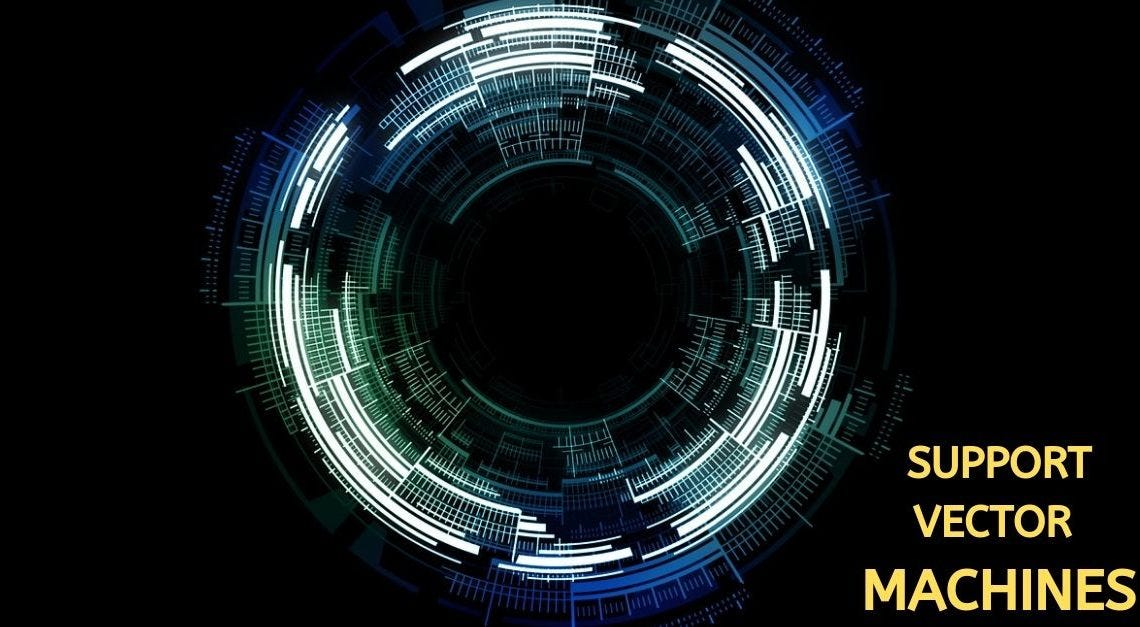Pic Credit: analyticsprofile

Machine Learning is considered as a subfield of Artificial Intelligence and it is concerned with the development of techniques and methods which enable the computer to learn. In simple terms development of algorithms which enable the machine to learn and perform tasks and activities. Machine learning overlaps with statistics in many ways. Over a period of time, many techniques and methodologies were developed for machine learning tasks.

In this article, we are going to learn almost everything about one such supervised machine learning algorithm which can be used for both classification and regression(SVR) i.e. Support Vector Machine or simply SVM. We’ll be focusing on classification in this article.

### Introduction

Support Vector Machines(SVM) are among one of the most popular and talked about machine learning algorithms.

They were extremely popular around the time they were developed in the 1990s and continue to be the go-to method for a high-performing algorithm with a little tuning.

The objective of SVM is to find a hyperplane in an N-dimensional space (N-Number of features) that distinctly classifies the data points.

Support Vector Machine is a generalization of maximal margin classifier. This classifier is simple, but it cannot be applied to the majority of the datasets since the classes must be separated by a boundary which is linear. But it does explain how the SVM works.

In the context of support-vector machines, the optimally separating hyperplane or maximum-margin hyperplane is a hyperplane which separates two convex hulls of points and is equidistant from the two.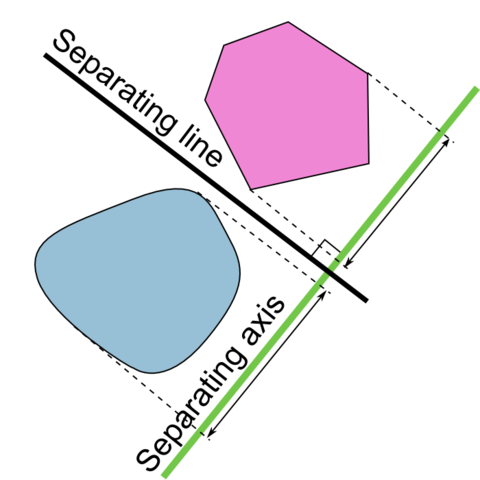Ok. What is a hyperplane?

In an N-dimensional space, a hyperplane is a flat affine subspace of dimension N-1. Visually, in a 2D space, a hyperplane will be a line and in 3D space, it will be a flat plane.

In simple terms, hyperplane is a decision boundary that helps classifying data points.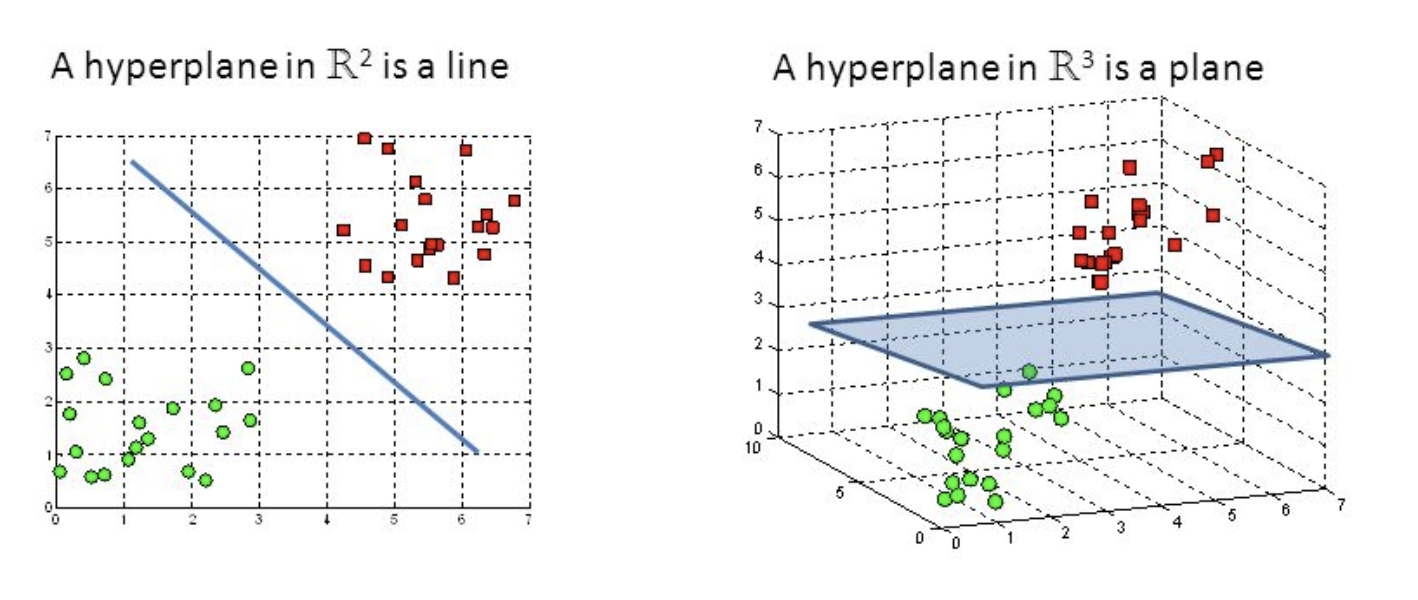Now, to separate two classes of data points, there are many possible hyperplanes that could be chosen. Our objective is to find a plane that has the maximum margin i.e. the maximum distance between data points of both classes and below figure clearly explains this fact.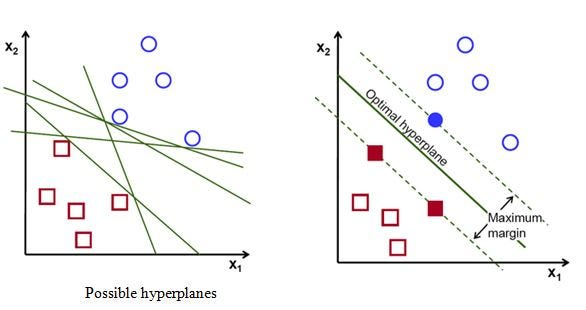Hyperplane with maximum margin looks something like this in 3D space: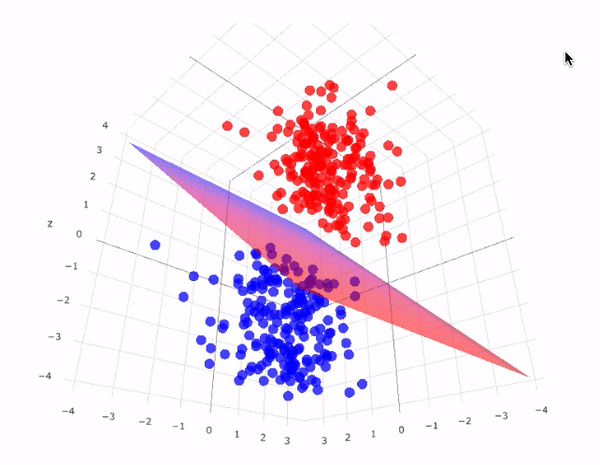Note:- The dimension of Hyperplane depends on the number of features.

Support Vectors

Support Vectors are the data points that are on or closest to the hyperplane and influence the position and orientation of the hyperplane. Using these support Vectors we maximize the margin of the classifier and deleting these support vectors will change the position of the hyperplane. These are actually the points that help us build SVM.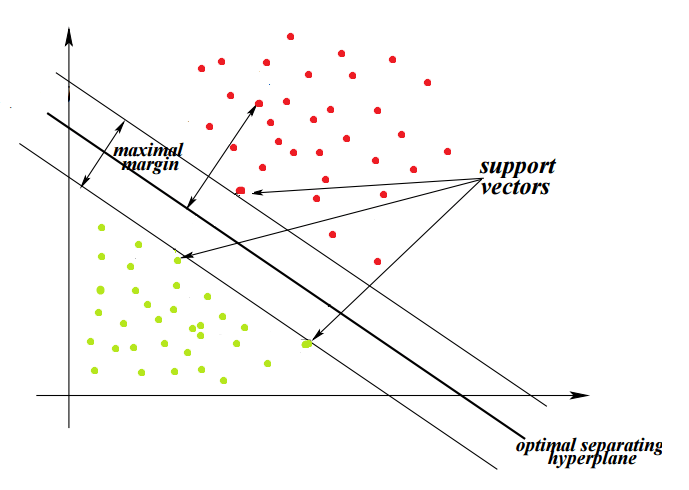Support Vectors are equidistant from the hyperplane. They are called support vectors because if their position shifts, the hyperplane shifts as well. This means that the hyperplane depends only on the support vectors and not on any other observations.

SVM that we have discussed until now can only classify the data which is linearly separable.

What if the data is non-linearly separated?

For example: look at the below image where the data is non-linearly separated, of course, we cannot draw a straight line to classify the data points.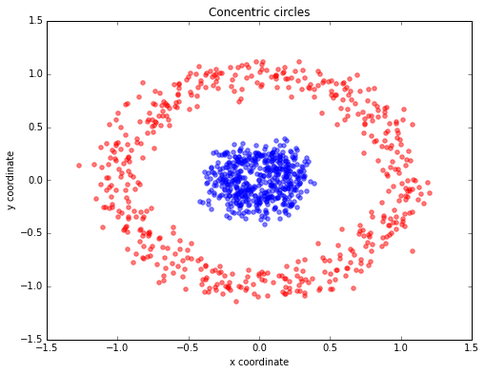Here comes the concept of Kernel in SVM to classify non-linearly separated data. A kernel is a function which maps a lower-dimensional data into higher dimensional data.

There are two ways by which kernel SVM will classify non-linear data.

1. Soft margin
2. Kernel tricks

Soft Margin

It allows SVM to make a certain number of mistakes and keep the margin as wide as possible so that other points can still be classified correctly.

“In other words, SVM tolerates a few dots to get misclassified and tries to balance the tradeoff between finding the line that maximizes the margin and minimizes misclassification.”

There are two types of misclassifications can happen:

1. The data point is on the wrong side of the decision boundary but on the correct side
2. The data point is on the wrong side of the decision boundary and on the wrong side of the margin

Degree of tolerance

How much tolerance we want to set when finding the decision boundary is an important hyper-parameter for the SVM (both linear and nonlinear solutions). In Sklearn, it is represented as the penalty term — ‘C’.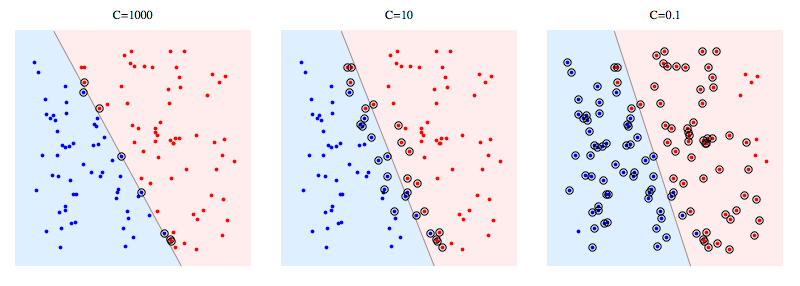The bigger the C, the more penalty SVM gets when it makes misclassification. Therefore, the narrower the margin is and fewer support vectors the decision boundary will depend on.

Kernel Trick

The idea is mapping the non-linear separable data from a lower dimension into a higher dimensional space where we can find a hyperplane that can separate the data points.

So it is all about finding the mapping function that transforms the 2D input space into a 3D output space and to reduce the complexity of finding the mapping function SVM uses Kernel Functions.

Kernel Functions are generalized functions that take 2 vectors(of any dimension) as input and output a score(dot product) that denotes how similar the input vectors are. If the dot product is small, vectors are different and if the dot product is large, vectors are more similar.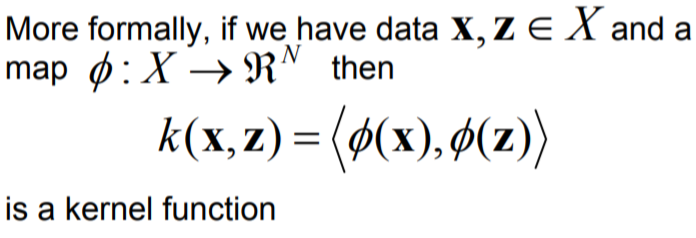Mathematical representation of Kernel Function

Pictorial representation of Kernel Trick :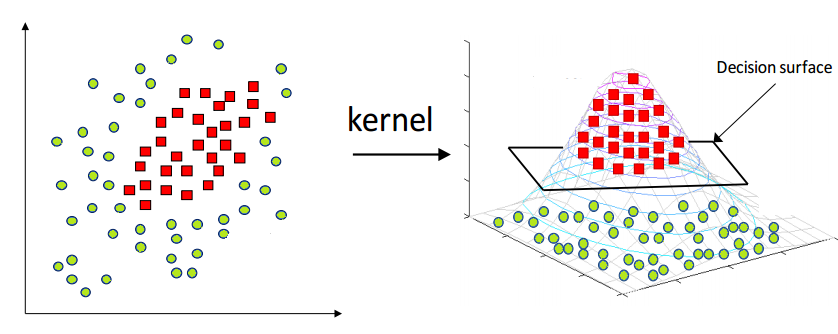Visual representation of Kernel Trick :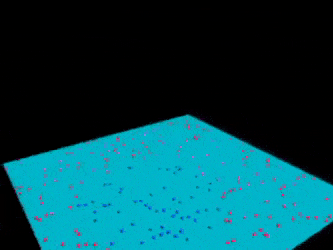Types of Kernel Functions:

1. Linear
2. Polynomial
4. Sigmoid

Let's talk about the most used kernel function i.e. Radial Basis Function(rbf).

Think of rbf as a transformer/processor to generate new features of higher dimension by measuring the distance between all other data points to a specific dot.

The most popular rbf kernel is Gaussian Radial Basis function. Mathematically: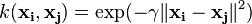where gamma(𝞬) controls the influence of new features on the decision boundary. Higher the value of 𝞬, more influence of features on the decision boundary.

Similar to Regularization parameter/penalty term(C) in the soft margin, Gamma(𝞬) is a hyperparameter that can be tuned when we use kernel trick.

### Conclusion

SVM has many uses ranging from face detection, image classification, Bioinformatics, Protein fold, and remote homology detection, handwriting recognition, generalized Predictive control(GPC), etc.

That's all for this article on Support Vector Machine which is one of the most powerful algorithms for both regression and classification. In the next article, we’ll see how to solve a real-world problem using SVM.

Bio: Nagesh Singh Chauhan is a Data Science enthusiast. Interested in Big Data, Python, Machine Learning.

Original. Reposted with permission.

Related:

Top Stories Past 30 DaysGet KDnuggets, a leading newsletter on AI, Data Science, and Machine Learning